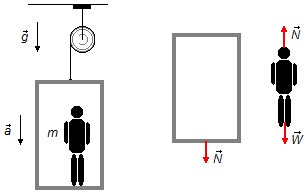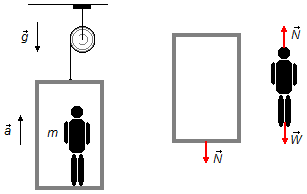Solved Problem on DynamicsPortuguêsEnglish

A man, of mass m = 70 kg, is in an elevator, this one moves with acceleration a = 2 m/s2 find:
a) The force of the man on the floor of the elevator, if the elevator is accelerating downwards;
b) The force of the man on the floor of the elevator, if the elevator is accelerating upwards;
c) For which acceleration of the elevator, will the force of the man on the floor will be equal to zero?
Assume free-fall acceleration g = 10 m/s2.

Problem data
• mass of the man:    m = 70 kg;
• acceleration of the elevator:    a = 2 m/s2;
• free-fall acceleration:    g = 10 m/s2}.
Solution
a) We choose the acceleration in the direction in which the elevator moves downward as positive.
Drawing a free-body diagram for the elevator and the man (figure 1) and applying Newton's Second Law
$\bbox[#99CCFF,10px] {\vec{F}=m\vec{a}} \tag{I}$
Elevator
• $$\vec{N}$$: normal force of the man on the floor of the elevator.
Mam
• $$\vec{W}$$: weight of the man;
• $$\vec{N}$$: normal force of the elevator on the man.figure 1

Note: In the elevator, there is also the weight of the elevator itself, but as we wish to find the force that man makes on the elevator and this is equal in intensity to the normal of the elevator on the man ($$\vec{N}$$), it is sufficient to analyze the forces acting on the man.

Using the expression (I) to man, we have
$W-N=ma$
the weight of the man is given by
$W=mg$
substituting this expression
$mg-N=ma\\ N=mg-ma$
factoring the mass m on the right side
$N=m\;(\;g-a\;) \tag{II}$
substituting the values given in the problem, we have
$N=70\times(\;10-2\;)\\ N=70\times8$
$\bbox[#FFCCCC,10px] {N=560\;\text{N}}$
b) We choose the acceleration in the direction in which the elevator moves upward as positive.
Drawing a free-body diagram for the elevator and the man and using Newton's Second Law.
The forces acting on the elevator and the man are the same as in item (a), figures 1 and 2.
Using the expression (I) to the man, with the inversion in the direction of the acceleration of the elevator we have
$N-W=ma$
using the same definition of weight of item (a)
$N-mg=ma\\ N=mg+ma$figure 2
factoring the mass m on the right side, we have
$N=m\;(\;g+a\;)$
substituting the values given in the problem, we have
$N=70\times(\;10+2\;)\\ N=70\times12$
$\bbox[#FFCCCC,10px] {N=840\;\text{N}}$

c) If the man does not exert force on the floor of the elevator we have the normal force null ($$N=0$$), substituting this condition in the expression (II) of item (a), we have
$0=m\;(\;g-a\;)\\ g-a=\frac{0}{m}\\ g-a=0$
$\bbox[#FFCCCC,10px] {a=g=10\;\text{m/s}^{2}}$
Note 1: This result means that the elevator and the man are in free fall, the man tends to "take off" from the elevator floor, there is no action of the man on the elevator and therefore there is no normal force on the man due to the elevator.

Note 2: If instead of using the expression (II) of the item (a) for the calculation we had used the expression $$N\;=\;m\;(\;g+a\;)$$ of item (b) with the condition of $$N\;=\;0$$, the result would be   $$a\;=\;-g$$. This means that the acceleration intensity would be the same, but the direction of acceleration, which in item (b) is pointed upwards, would have to be downwards, which would coincide with the situation of item (a).